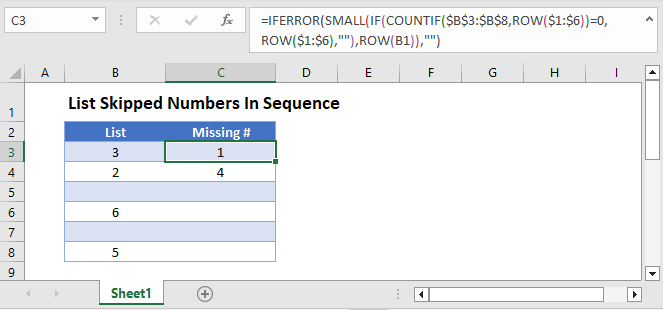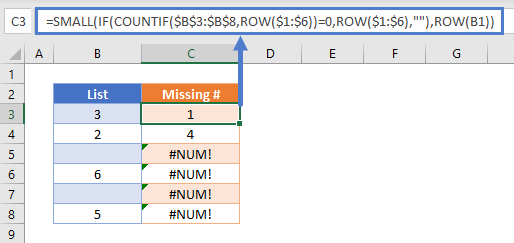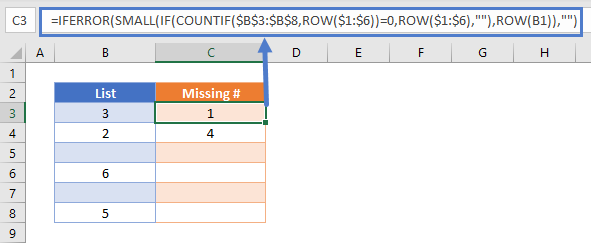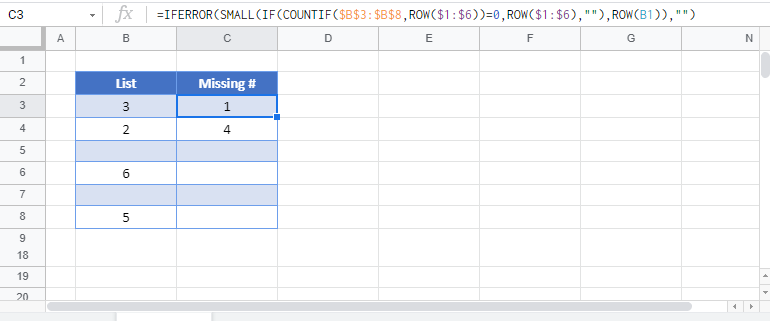# List Skipped Numbers in Sequence – Excel & Google Sheets

This tutorial will demonstrate how to list missing numbers in a range.## List Skipped Numbers in Sequence

To find missing numbers in a list, we can use the following formula:

``=SMALL(IF(COUNTIF(\$B\$3:\$B\$8,ROW(\$1:\$6))=0,ROW(\$1:\$6),""),ROW(B1))``Notes:

• This is an array formula! When using Excel 2019 and earlier, you must enter the formula by pressing CTRL + SHIFT + ENTER. You’ll know the formula was entered properly when you see curly brackets around the formula.
• This formula only works with positive whole numbers (integers).

## How does the formula work?

First, the ROW Function is used to generate an array of numbers:

``{1;2;3;4;5;6}``

In fact, you could even enter in this array of numbers manually:

``=SMALL(IF(COUNTIF(\$B\$3:\$B\$8,{1;2;3;4;5;6})=0,{1;2;3;4;5;6},""),ROW(B1))``

Next, The COUNTIF Function will count the values “if” they match a value found in the array.

To visualize this, first we will convert the range \$B\$3:\$B\$8 to it’s array of values {3;2;0;6;0;5}:

``=SMALL(IF(COUNTIF({3;2;0;6;0;5},{1;2;3;4;5;6})=0,{1;2;3;4;5;6},""),ROW(B1))``

Next we will perform the COUNTIF operation:

``=SMALL(IF({0;1;1;0;1;1}=0,{1;2;3;4;5;6},""),ROW(B1))``

If a value is not found 0 is outputted. The IF Function checks if the value is NOT Found and if so, returns the value from the array, otherwise it outputs blank:

``=SMALL({1;"";"";4;"";""},ROW(B1))``

Last, the SMALL Function returns the smallest missing value in the selected cell.

``=SMALL({1;"";"";4;"";""},{1})``
``={1}``

Tip:

To avoid the #NUM! error, wrap your formula with the IFERROR Function:

``=IFERROR(SMALL(IF(COUNTIF(B3:B8,ROW(1:6))=0,ROW(1:6),""),ROW(B1)),"")``## List Skipped Numbers in Sequence in Google Sheets

All the examples explained above work the same in Google sheets as they do in Excel.### Excel Practice Worksheet

Practice Excel functions and formulas with our 100% free practice worksheets!